# Mr. R.’s World of Math

## Triangle Types

### how many types of triangles do you know?

Types of triangles?
More than 5!
Have three angles,
Have three sides…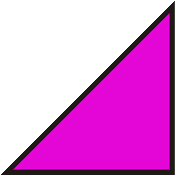Right ones first,
Pythagoras knows,
All right triangles,
Fabulous those!
Now we’ve started,
Getting loose,
Right tri’s long side?
Hypotenuse!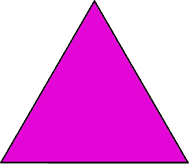Equilateral?
Sides the same,
Angles equal,
It’s their game!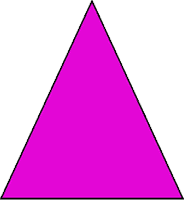Isosceles?
2 equal sides,
2 equal angles,
Playground slides!Scalene triangles,
Have one catch,
Different sides,
No angles match!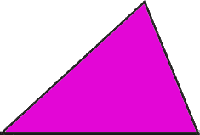Acute triangles?
Sides in threes,
All angles less,
Than 90º,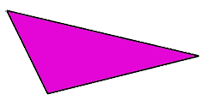Obtuse triangle?
Angle measure,
More than 90,
What a pleasure…
Those are 6,
Types of tri’s
Have 3 angles, have 3 sides…

RELATED MATH CHALLENGES

If a right triangle has angles of 90 and 35 degrees, what is the third angle of the triangle?
Can a scalene triangle have two angles that are the same measure?
Can an obtuse triangle have two angle measures that are 45 degrees each? Why or why not?

RELATED MATH MUSIC VIDEOSRELATED MATH SONGS